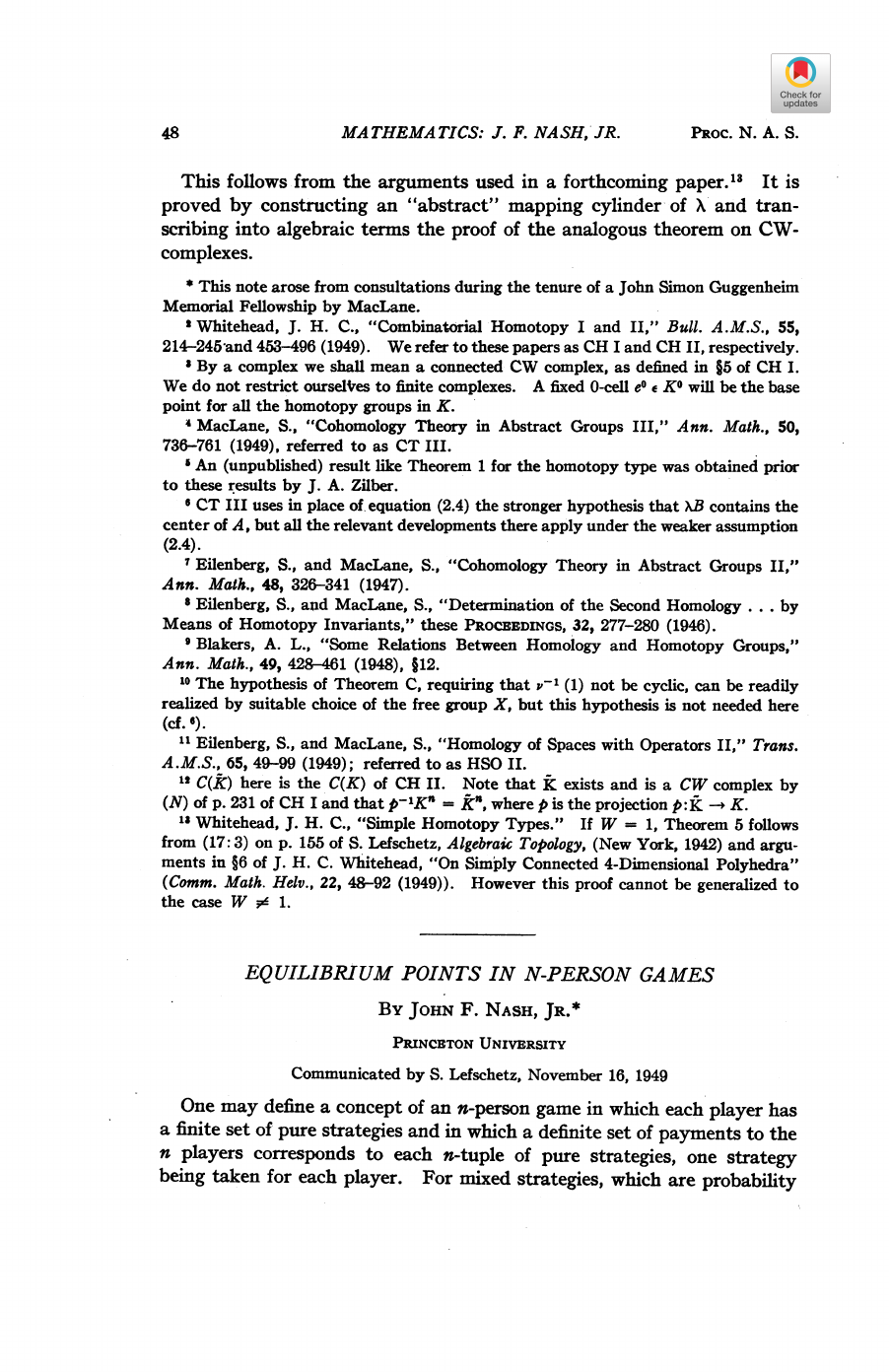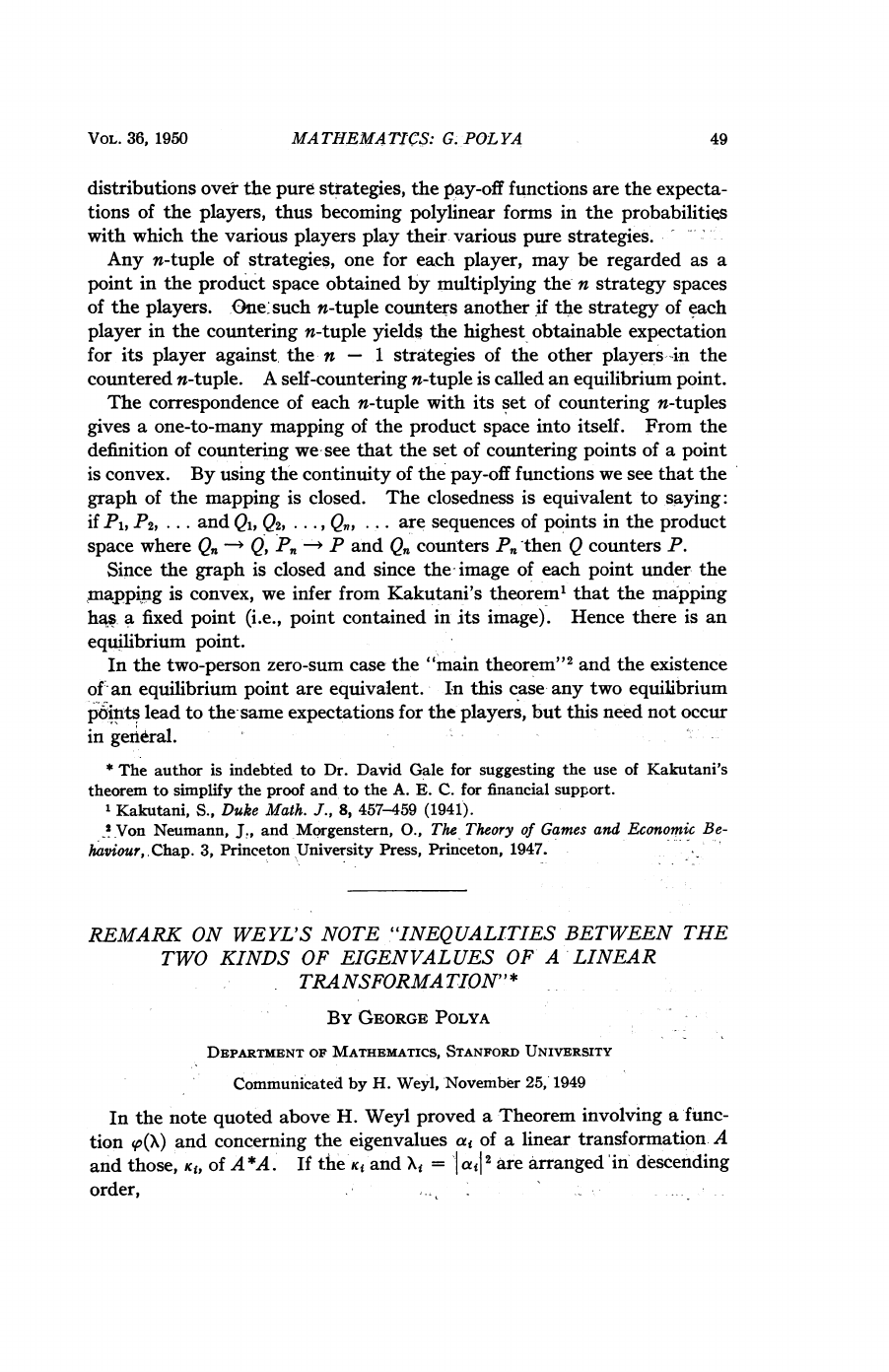John Forbes Nash Jr. was an American mathematician and ***the only ...
### TL;DR This short paper published in 1949 is one of the mos...
John Nash was a mathematical genius. He is the only person to be aw...
**Fun fact:** The movie ["A Beautiful Mind"](https://www.imdb.co...
Does these n-tuple converges ?
Here is a brief lecture that gives an intuitive explanation of the ...
The ***Prisoner's dilemma*** in game theory is a special manifestat...MA
THEMA
TICS:
J.
F.
NASH,
JR.
This
follows
from
the
arguments
used
in
a
forthcoming
paper."'
It
is
proved
by
constructing
an
"abstract"
mapping
cylinder
of
X
and
tran-
scribing
into
algebraic
terms
the
proof
of
the
analogous
theorem
on
CW-
complexes.
*
This
note
arose
from
consultations
during
the
tenure
of
a
John
Simon
Guggenheim
Memorial
Fellowship
by
MacLane.
'
J.
H.
C.,
"Combinatorial
Homotopy
I
and
II,"
Bull.
A.M.S.,
55,
214-245
and
453-496
(1949).
We
refer
to
these
papers
as
CH
I
and
CH
II,
respectively.
'
By
a
complex
we
shall
mean
a
connected
CW
complex,
as
defined
in
§5
of
CH
I.
We
do
not
restrict
ourselres
to
finite
complexes.
A
fixed
0-cell
e
KO
will
be
the
base
point
for
all
the
homotopy
groups
in
K.
4
MacLane,
S.,
"Cohomology
Theory
in
Abstract
Groups
III,"
Ann.
Math.,
50,
736-761
(1949),
referred
to
as
CT
III.
'
An
(unpublished)
result
like
Theorem
1
for
the
homotopy
type
was
obtained
prior
to
these
results
by
J.
A.
Zilber.
CT
III
uses
in
place
of.
equation
(2.4)
the
stronger
hypothesis
that
XB
contains
the
center
of
A,
but
all
the
relevant
developments
there
apply
under
the
weaker
assumption
(2.4).
I
Eilenberg,
S.,
and
MacLane,
S.,
"Cohomology
Theory
in
Abstract
Groups
II,"
Ann.
Math.,
48,
326-341
(1947).
8
Eilenberg,
S.,
and
MacLane,
S.,
"Determination
of
the
Second
Homology
...
by
Means
of
Homotopy
Invariants,"
these
PROCEEDINGS,
32,
277-280
(1946).
9
Blakers,
A.
L.,
"Some
Relations
Between
Homology
and
Homotopy
Groups,"
Ann.
Math.,
49,
428-461
(1948),
§12.
10
The
hypothesis
of
Theorem
C,
requiring
that
v-
(1)
not
be
cyclic,
can
be
realized
by
suitable
choice
of
the
free
group
X,
but
this
hypothesis
is
not
needed
here
(cf.
6).
11
Eilenberg,
S.,
and
MacLane,
S.,
"Homology
of
Spaces
with
Operators
II,"
Trans.
A.M.S.,
65,
49-99
(1949);
referred
to
as
HSO
II.
12
C(k)
here
is
the
C(K)
of
CH
II.
Note
that
K
exists
and
is
a
CW
complex
by
(N)
of
p.
231
of
CH
I
and
that
p-'K'
-
K",
where
p
is
the
projection
p:K
--
K.
13
J.
H.
C.,
"Simple
Homotopy
Types."
If
W
=
1,
Theorem
5
follows
from
(17:3)
on
p.
155
of
S.
Lefschetz,
Algebraic
Topology,
(New
York,
1942)
and
argu-
ments
in
§6
of
J.
H.
C.
"On
Simply
Connected
4-Dimensional
Polyhedra"
(Comm.
Math.
Helv.,
22,
48-92
(1949)).
However
this
proof
cannot
be
generalized
to
the
case
W
#
1.
EQUILIBRIUM
POINTS
IN
N-PERSON
GAMES
By
JOHN
F.
NASH,
JR.*
PRINCETON
UNIVERSITY
Communicated
by
S.
Lefschetz,
November
16,
1949
One
may
define
a
concept
of
an
n-person
game
in
which
each
player
has
a
finite
set
of
pure
strategies
and
in
which
a
definite
set
of
payments
to
the
n
players
corresponds
to
each
n-tuple
of
pure
strategies,
one
strategy
being
taken
for
each
player.
For
mixed
strategies,
which
are
probability
PROC.
N.
A.
S.
48MA
THEMATICS:
G.
POL
YA
distributions
over
the
pure
strategies,
the
pay-off
functions
are
the
expecta-
tions
of
the
players,
thus
becoming
polylinear
forms
in
the
probabilities
with
which
the
various
players
play
their
various
pure
strategies.
Any
n-tuple
of
strategies,
one
for
each
player,
may
be
regarded
as
a
point
in
the
product
space
obtained
by
multiplying
the-
n
strategy
spaces
of
the
players.
One:-such
n-tuple
counters
another
if
the
strategy
of
each
player
in
the
countering
n-tuple
yields
the
highest
obtainable
expectation
for
its
player
against,
the
n
-
1
strategies
of
the
other
players
in
the
countered
n-tuple.
A
self-countering
n-tuple
is
called
an
equilibrium
point.
The
correspondence
of
each
n-tuple
with
its
set
of
countering
n-tuples
gives
a
one-to-many
mapping
of
the
product
space
into
itself.
From
the
definition
of
countering
we-see
that
the
set
of
countering
points
of
a
point
is
convex.
By
using
the
continuity
of
the
pay-off
functions
we
see
that
the
graph
of
the
mapping
is
closed.
The
closedness
is
equivalent
to
saying:
if
Pi,
P2,
...
and
Qi,
Q2,
....
Qn,
...
are
sequences
of
points
in
the
product
space
where
Q.
-n
Q,
P
n
P
and
Q,,
counters
P,,
then
Q
counters
P.
Since
the
graph
is
closed
and
since
the
-image
of
each
point
under
the
mapping
is
convex,
we
infer
from
Kakutani's
theorem'
that
the
mapping
has
a
fixed
point
(i.e.,
point
contained
in
its
image).
Hence
there
is
an
equilibrium
point.
In
the
two-person
zero-sum
case
the
"main
theorem"2
and
the
existence
of,
an
equilibrium
point
are
equivalent.
In
this
case
any
two
equilibrium
points
to
the-same
expectations
for
the
players,
but
this
need
not
occur
in
general.
*
The
author
is
indebted
to
Dr.
David
Gale
for
suggesting
the
use
of
Kakutani's
theorem
to
simplify
the
proof
and
to
the
A.
E.
C.
for
financial
support.
'Kakutani,
S.,
Duke
Math.
J.,
8,
457-459
(1941).
2
Von
Neumann,
J.,
and
Morgenstern,
O.,
The
Theory
of
Games
and
Economic
Be-
haviour,
Chap.
3,
Princeton
University
Press,
Princeton,
1947.
REMARK
ON
WEYL'S
NOTE
"INEQUALITIES
BETWEEN
THE
TWO
KINDS
OF
EIGENVALUES
OF
A
LINEAR
TRANSFORMATION"
*
By
GEORGE
POLYA
DEPARTMENT
OF
MATHEMATICS,
STANFORD
UNIVERSITY
Communicated
by
H.
Weyl,
November
25,
1949
In
the
note
quoted
above
H.
Weyl
proved
a
Theorem
involving
a
func-
tion
so(X)
and
concerning
the
eigenvalues
aj
of
a
linear
transformation
A
and
those,
Ki,
of
A*A.
If
the
Kj
and
xi
=
IaiI2
are
arranged
in
descending
order,
VOL.
36,
1950
49